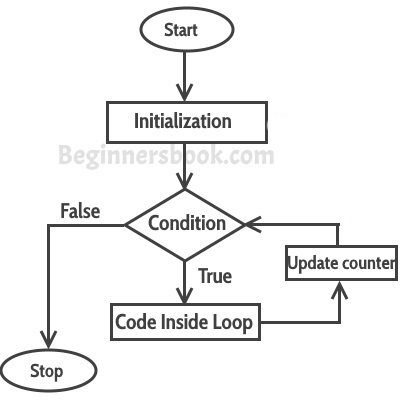World's most popular travel blog for travel bloggers.

# Loop Control Statement : For Loop

, ,

## For Loop

Loops are used to execute a set of statements repeatedly until a particular condition is satisfied. In Java we have three types of basic loops: for, while and do-while. In this tutorial we will learn how to use “for loop” in Java.

#### Syntax of for loop:

```for(initialization; condition ; increment/decrement)
{
statement(s);
}```

## Flow of Execution of the for Loop

As a program executes, the interpreter always keeps track of which statement is about to be executed. We call this the control flow, or the flow of execution of the program.First step: In for loop, initialization happens first and only one time, which means that the initialization part of for loop only executes once.
Second step: Condition in for loop is evaluated on each iteration, if the condition is true then the statements inside for loop body gets executed. Once the condition returns false, the statements in for loop does not execute and the control gets transferred to the next statement in the program after for loop.
Third step: After every execution of for loop’s body, the increment/decrement part of for loop executes that updates the loop counter.
Fourth step: After third step, the control jumps to second step and condition is re-evaluated.

## Example of Simple For loop

```class ForLoopExample {
public static void main(String args[]){
for(int i=10; i>1; i--){
System.out.println("The value of i is: "+i);
}
}
}```
The output of this program is:
```The value of i is: 10
The value of i is: 9
The value of i is: 8
The value of i is: 7
The value of i is: 6
The value of i is: 5
The value of i is: 4
The value of i is: 3
The value of i is: 2```
In the above program:
int i=1 is initialization expression
i>1 is condition(Boolean expression)
i– Decrement operation

## Infinite for loop

The importance of Boolean expression and increment/decrement operation co-ordination:
```class ForLoopExample2 {
public static void main(String args[]){
for(int i=1; i>=1; i++){
System.out.println("The value of i is: "+i);
}
}
}```
This is an infinite loop as the condition would never return false. The initialization step is setting up the value of variable i to 1, since we are incrementing the value of i, it would always be greater than 1 (the Boolean expression: i>1) so it would never return false. This would eventually lead to the infinite loop condition. Thus it is important to see the co-ordination between Boolean expression and increment/decrement operation to determine whether the loop would terminate at some point of time or not.
Here is another example of infinite for loop:
```// infinite loop
for ( ; ; ) {
// statement(s)
}```

#### Post a Comment

Let us know your responses and feedback